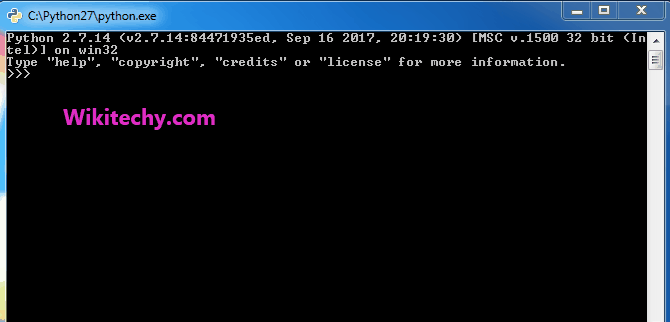# python tutorial - Logical Operators | Logical Operators on String in Python - learn python - python programming

For strings in python, boolean operators (and , or) work. Let us consider the two strings namely str1 and str2 and try boolean operators on them:Learn Python - Python tutorial - python striprepr - Python examples - Python programs

python - Sample - python code :

Output:

The output of the boolean operations between the strings depends on following things:

1. Python considers empty strings as having boolean value of ‘false’ and non-empty string as having boolean value of ‘true’.
2. For ‘and’ operator if left value is true, then right value is checked and returned. If left value is false, then it is returned
3. For ‘or’ operator if left value is true, then it is returned, otherwise if right value is false, then right value is returned.

Note that the bitwise operators (| , &) don’t work for strings.

Wikitechy tutorial site provides you all the learn python , python course online free , free python training , python programming learning , free python course